Spectral homology

(diff) ← Older revision | Latest revision (diff) | Newer revision → (diff)

The inverse limit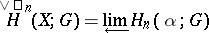of homology groups with coefficients in the Abelian groupof nerves of open coveringsof a topological space(also called Čech homology, or Aleksandrov–Čech homology). For a closed set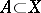, the groups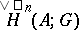can be defined in a similar way using the subsystems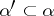of all those subsets ofhaving non-empty intersection with. The inverse limit of the groups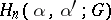is called the spectral homology groupof the pair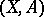.

Since the inverse limit functor does not preserve exactness, the homology sequence of the pairis, in general, not exact. It is semi-exact, in the sense that the composite of any two mappings in the sequence is equal to zero. For a compact spacethe sequence turns out to be exact in the case whenis a compact group or field (or, more generally, ifis algebraically compact). The spectral homology of compact spaces is continuous in the sense that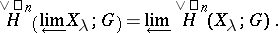Lack of exactness is not the only deficiency of spectral homology. The groups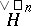turn out to be non-additive, in the sense that the homology of a discrete union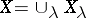can be different from the direct sum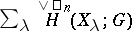. This deficiency disappears if one considers the spectral homology groupswith compact support, defined as the direct limit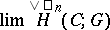taken over all compact subsets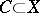. It is natural to consider the functor, in view of the fact that all the usual homologies (simplicial, cellular and singular) are homologies with compact support.

The difference between the functorsandis one of the examples of how homology groups react to small changes in their initial definition (on the other hand, cohomology groups exhibit significant stability in this respect). Among the logically possible variants of the definition of homology groups in general categories of topological spaces, the correct one was not the first to be selected. The theory of the homology groupsassociated with the Aleksandrov–Čech cohomology achieved great recognition only in the 1960's (although the first definitions were given in the 1940's and 1950's). The theory of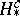satisfies all the Steenrod–Eilenberg axioms (and is a theory with compact supports). For compact spacesthe following sequence is exact: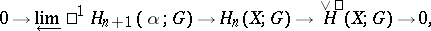where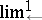is the derived inverse limit functor. In general there is an epimorphism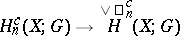whose kernel is zero for any algebraically compact group. For any locally compact space that is also homologically locally connected (with respect to), the functors,,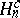are isomorphic.

How to Cite This Entry:
Spectral homology. Encyclopedia of Mathematics. URL: http://encyclopediaofmath.org/index.php?title=Spectral_homology&oldid=17746
This article was adapted from an original article by E.G. Sklyarenko (originator), which appeared in Encyclopedia of Mathematics - ISBN 1402006098. See original article